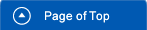Home > Various Aspects > Inclination of High-rise Towers due to Winds## Inclination of High-rise Towers due to Winds

Building semi-floating water cities that is capable of supporting numerous high-rise towers would mitigate the land shortage while providing a means of coping with Singapore’s growing population. However, ensuring the stability of a floating water city is a fundamental safety requirement, especially when considering the construction of high-rise buildings on floating foundations. Therefore, calculations related to the static stability and inclination of high-rise towers subject to the highest wind intensity levels are provided in the following paragraphs.Fig.1 Perspective View of Proposed High-density Water City in Singapore

The total weight of three 51-story high-rise towers, each 210 m high and 60 m wide (as shown in Figs. 2), is estimated as 529,200 tf by assuming a unit weight of 1.2 tf/m2. The deadweight of the sum of three floating units (each unit having a footprint of 135 m by 135 m, and being composed of a floating foundation and a building) is 146,880 tf based on the specific weight of 0.167 tf/m3 that was obtained from the “Mega-float” project. It follows that the final total weight will be the sum of these dead weights of 676,080 tf, plus the variable weight estimated as 39,420 tf. The total underwater volume would be 715,500 m3.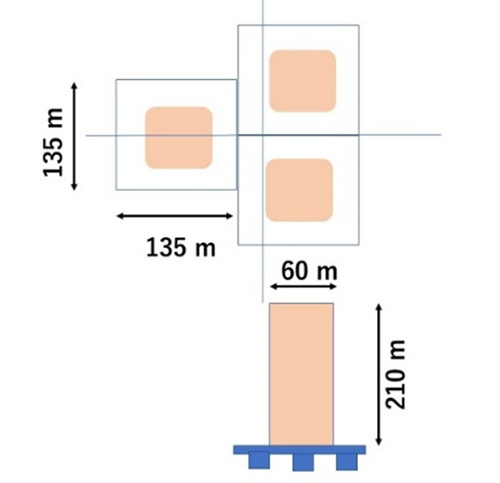Fig.2 Schematic Plan and Vertical Views of Floating Foundations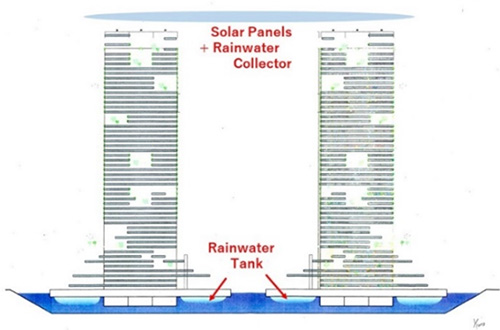Fig.3 Vertical View of Semi-floating Water City

The location of the metacenter (BM), which is defined as the vertical distance between the center of buoyancy and the metacenter of the floating units, is estimated by the following equation: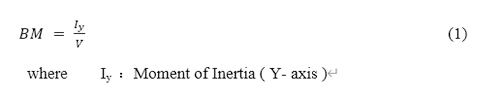In general, a moment of inertia for a floating unit of rectangular shape is calculated by Equation 2 below. Here, b=L in this case.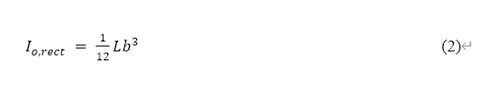Then the final values of both BM and the GM (vertical distance between the center of gravity and the metacenter) for a floating foundation composed of three floating units are calculated as 348,2 m and 257.5 m, respectively, while the vertical location of the center of gravity of these floating system is 103.3 m.

Next, assuming a maximum wind intensity of 60 m/s at 105 m from the water level, the total wind force on the three super-tall buildings on a floating foundation is calculated as 163,976.4 kN by assuming a drag coefficient of 2.0. Furthermore, the corresponding total wind heeling moment is calculated as 17,217,522 kN･m.

Assuming the wind heeling moment Ms is the same as the restoring moment MR of the floating units, the following equation is produced: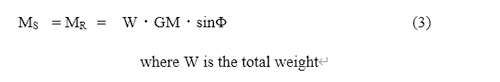Next, the inclination of the floating units Φ due to wind can be estimated by the following equation: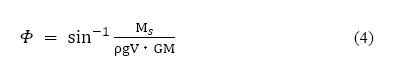Finally, the inclination due to a 60 m/s wind at 105 m above the water level is estimated to be 0.55, which is considered sufficiently low and stable.